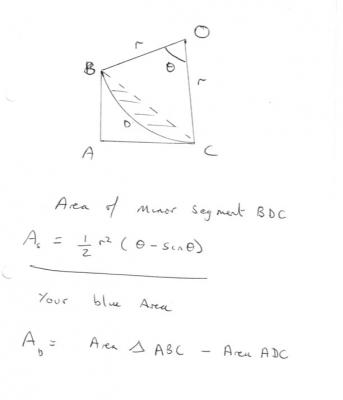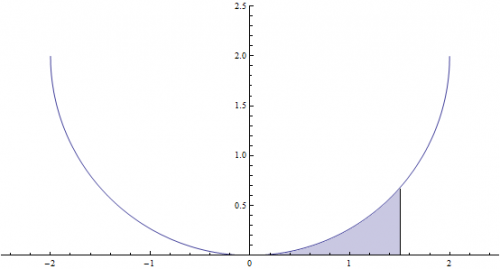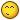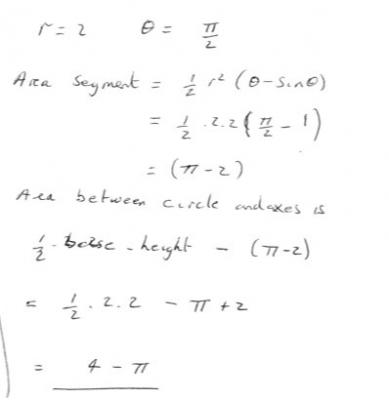# Finding the Area Under a Circle Using Integrals?

## Recommended Posts

How would I find the area shaded in blue below? (please forgive how big the image is)

I'm assuming you'd have to use calculus as there is no way to simply subtract the area of the circle from the area of a larger rectangle without ending up looking for a limit.

I guess I don't know how I'd go about finding the anti-derivative of a circle.#### Share this post

##### Share on other sites

Given the equation for a circle offset from the origin such that the bottom of the circle touches the $x$ axis we get:

$x^2+(y-r)^2=r^2$

Solving for $y$, we get:

$(y-r)^2=r^2-x^2$

$y-r=\sqrt{r^2-x^2}$

$y=\sqrt{r^2-x^2}+r$

Because square roots are involved, there are two functions to consider. The top half of the circle:

$y=r+\sqrt{r^2-x^2}$

or the bottom half:

$y=r-\sqrt{r^2-x^2}$

Since your diagram shows the bottom half, we'll go with

$f(x)=r-\sqrt{r^2-x^2}$

Now, we simply integrate the function on a specified interval [$a,b$]

$\int_{a}^{b}r-\sqrt{r^2-x^2}\, dx$

or, we can solve for the antiderivative to get:

$\int r-\sqrt{r^2-x^2}\, dx = r x - \frac{1}{2}\, x\, \sqrt{r^2 - x^2} + \frac{1}{2}\, r^2\, \text{tan}^{-1}\left (\frac{x \sqrt{r^2 - x^2}}{x^2-r^2}\right )+C$

Edited by Daedalus

#### Share this post

##### Share on other sites

A much simpler geometric (non calculus) method is to subtract the area of the minor segment of the circle from the area of the right triangle as shown in my diagram.#### Share this post

##### Share on other sites

In studiot's solution;

The required area is area of ∆ABC - area BDCB.#### Share this post

##### Share on other sites

So it is.

Thanks for correcting my oversight. +1

You are generous with upvotes, Sir.#### Share this post

##### Share on other sites

Not answering the question, about integrals, but solving the blue area question, one could take a geometric approach.

The radius of circle is two units and its area can be calculated by pi r squared.

The area of the square that encompasses the circle is 16.

The difference between these two areas is the area that is within the square and outside the circle.

One quarter of this difference is between the circle and the axis.

Then, looking at this area you see three areas. One in blue, another the same shape as the blue invisible up agaist the y axis, and a third area which looks from the scale of the drawing, as if it might be a square, drawable as if .5 x and .5 y would be a point on the circle. If this is true, which we could check by seeing if the difference between the diagonal of a .5 by .5 square and a 2 by 2 square is 1, then the problem would be solved by taking away .25 from the area we figured is between the circle and the axis, and dividing the remainder by 2.

Regards, TAR

I meant to say 2 not 1, as the radius is 2 and 2 , which is the portion of diagonal from 2,2 to the origin within the circle, would leave a certain distance between the circle and the origin, which would be equal to the hypotenuse of a .5,.5 right triangle.

#### Share this post

##### Share on other sites

Actually, upon doing the check, its a .865 or so square, where the corner would be on the circle. So abandon my method.

Although there still is an integral problem to do with my method concerning that area between .5 and .586. That .086 wide slice that runs up from the x axis to the circle is the difference between the blue area had the problem stated .586 and the blue area as it is at .5. And so if you were to solve the problem the geometric way, as if it had stated .586, you would be close to the correct answer and you would just have to add the area of this slice, which is mostly rectangular at .5 x .086, with just a little area left under the curve, that could be figured with integration.

So anyway. Nevermind. My check did not work out. The square root of 8 is 2.8284, the square root of .5 squared plus .5 squared (.5) is .7071 and the difference between the two is 2.1213. Where we (I) needed it to be 2 for the method to work.

Sorry again, my edit is not working right. I typed .865 and meant to type .586.

All,

I get about .3116 using my method.

What do you get Daedalus? Studiot? metacogitans?

What is the answer?

Regards TAR

This isn't a homework question, is it?

#### Share this post

##### Share on other sites

What do you get Daedalus?

Using the integral, shifting the function as I did, and assuming a radius of 2, we end up with an interval [0, 1.5]. It is important to note that, even though the OP is integrating the left side of the circle, the symmetry of the function allows us to integrate either side as long as we adjust the limits of integration.Substituting these numbers into the integral yields:

$\int_{0}^{1.5}2-\sqrt{2^2-x^2}\, dx = 0.31171910038781636...$

Of course, the antiderivative gives the same result:

$(2)x - \frac{1}{2}\, x\, \sqrt{(2)^2 - x^2} + \frac{1}{2}\, (2)^2\, \text{tan}^{-1}\left (\frac{x \sqrt{(2)^2 - x^2}}{x^2-(2)^2}\right ) \Bigg|_{0}^{1.5} = 0.31171910038781636...$

(For those not familiar with trig functions, $\text{tan}^{-1} \theta = \text{arctan}\, \theta$)

So, the answer you got Tar is a good approximation.Edited by Daedalus

#### Share this post

##### Share on other sites

@Tar

For the quarter circle you describe

The pesky LaTex bug has struck again so here is a scan.#### Share this post

##### Share on other sites

Studiot, I think you messed up on the calculation because $4-\pi = 0.858407346410206... \ne 0.31171910038781636...$ .

Edited by Daedalus

#### Share this post

##### Share on other sites

Not answering the question, about integrals, but solving the blue area question, one could take a geometric approach.

The radius of circle is two units and its area can be calculated by pi r squared.

The area of the square that encompasses the circle is 16.

The difference between these two areas is the area that is within the square and outside the circle.

One quarter of this difference is between the circle and the axis.

Daedalus, I answered this question, which I think is not the same as the original since that was not for a quarter circle

#### Share this post

##### Share on other sites

Daedalus, I answered this question, which I think is not the same as the original since that was not for a quarter circle

I see... My bad. I thought you were providing the answer for the region being integrated.#### Share this post

##### Share on other sites

Studiot,

So anyway, what is your answer, by your triangle method, to the original blue area question?

Daedalus already gave us the exact answer, that you get through integration, my approximation using my method was close, and would have needed integration to get to the exact answer because I still had the little peice under the curve that I just had to approximate. What number do you get with your triangle method? That is, does your method work to get an exact answer, or is integration the only way to go?

Regards, TAR

#### Share this post

##### Share on other sites

No, I answered the general problem.

To specifically calculate metacogitans figures you need to calculate the value of y at x = 0.5, say Y, from the equation for the circle, to obtain a second side to the triangle.

The angle subtended at the centre is then twice the angle whose tangent is given by Y/X , or you can obtain the centre angle by calculating the chord and then using the cosine rule.

So the equation of a circle the circle is (x-a)2+(y-b)2=r2. In this case a = b = r = 2

Solving for y leads to the two values, we want the lower or smaller one of 0.67712434.

This leads to the tangent of the deflection angle (in radians) being 0.67712434/1.5 = 0.45141623

The deflection angle is thus 0.424031 radians

The angle at the centre (theta) is twice this or 0.848062 radians

The sine of this is 0.75

Thus the area of the segment is 2(0.848062-0.75) = 0.19724 area units.

The area of the triangle is 1/2 base x height = 0.5*1.5*0.677124 = 0.507843 area units

The difference is (0.507843 - 0.19724) = 0.311 area units.

This method is accurate for all values and does not require calculus.

Does this answer your question, Tar?

Edited by studiot

#### Share this post

##### Share on other sites

Studiot,

Nice, I didn't get it the first time.

So I hope that answers the OP.

We have the trig/geometry method. (Studiot)

The integral calculus/trig method. (Daedalus)

And the goofy, sort of geometry peicemeal get you close but requiring integration to finish method.(TAR)

Regards, TAR

## Create an account or sign in to comment

You need to be a member in order to leave a comment

## Create an account

Sign up for a new account in our community. It's easy!

Register a new account

## Sign in

Already have an account? Sign in here.

Sign In Now